Courses

Test: First Law of Thermodynamics - 2

15 Questions MCQ Test Thermodynamics | Test: First Law of Thermodynamics - 2

Description
Attempt Test: First Law of Thermodynamics - 2 | 15 questions in 45 minutes | Mock test for Mechanical Engineering preparation | Free important questions MCQ to study Thermodynamics for Mechanical Engineering Exam | Download free PDF with solutions
QUESTION: 1

According to first law of thermodynamics

Solution:

Since first law of thermodynamics defined as law of conservation of energy hence total energy of a system remains constant.

QUESTION: 2

Internal energy is defined by

Solution:

Zeroth law of thermodynamics — concept of temperature
First law of thermodynamics — concept of internal energy
Second law of thermodynamics — concept of entropy.

QUESTION: 3

Key concept in analyzing the filling of an evacuated tank is

Solution:
QUESTION: 4

First law of thermodynamics is valid for

Solution:
QUESTION: 5

During a thermodynamic process, 84 kJ of heat flows into the system and the work done by the system is 32 kJ. The increase in internal energy of the system is

Solution:

From first law of thermodynamics
δQ = dU+δW
84 = dU +32
dU = 52kJ

QUESTION: 6

The specific heat at constant pressure for an ideal gas is given by
cp = 0.9 + (2.7 x 10-4) T (kJ/kgK)
Where T is in kelvin. The change in enthalpy for this ideal gas undergoing a process in which the temperature changes from 27°C to 127°C is most nearly.

Solution: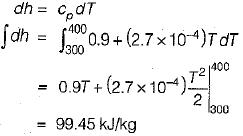QUESTION: 7

A closed system undergoes a process 1-2 for which the values of Q1-2 and W1-2 are +20 kJ and +50 kJ respectively. If the system is returned to state 1 and Q2-1 is - 10 kJ what is the value of work W2-1

Solution: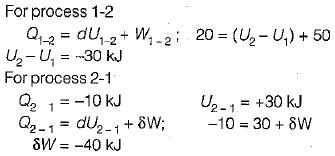QUESTION: 8

Given that along the path 1-2-3 a system absorbs 100 kJ as heat and does 60 kJ work while along the path 1 -4-3 it does 20 kJ work (see figure given). The heat absorbed during the cycle 1-4-3 is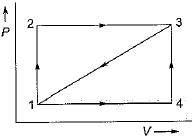Solution: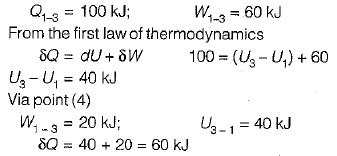QUESTION: 9

The network output for the cycle 1-2-3-4 -5-6-1 shown in figure is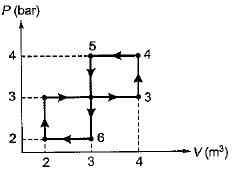Solution:
QUESTION: 10

In a reversible isothermal expansion process fluid expands from 10 bar and 2 m3 to 2 bar and 10 m3. During the process the heat supplied is at the rate of 100 kW. What is the rate of work done during the process

Solution:

Note that in case of reversible isothermal expansion change in internal energy is zero hence
δQ = δW= 100 kW

QUESTION: 11

The state of an ideal gas is changed from (T1, P1) to (T2, P2) in a constant volume process. To calculate the change in enthalpy (Δh) ail of the following properties/variables are required.

Solution: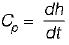QUESTION: 12

For the two paths as shown in the figure, one reversible and one irreversible, to change the state of the system from a to b.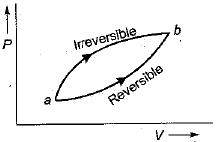Solution:

ΔU is a point function and it is independent of the path followed.

QUESTION: 13

The net work done for the closed system shown in the given pressure-volume diagram is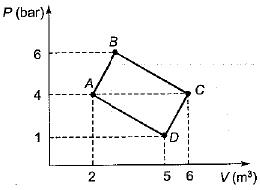Solution:

Explanation : AC = 4 m3.

ABC = 2, ACD = 3

Work done = Area of ABC + Area of ACD

= 1/2 * 2 * 4 + 1/2 * 3 * 4

= 1000 kN-m.

QUESTION: 14

Two ideal heat engine cycles are represented in the given figure. Assume VQ = QR, PQ = QS and UP = PR= RT. If the work interaction for the rectangular cycle (WVRU) is 48 Nm, then the work interaction for the other cycle PST is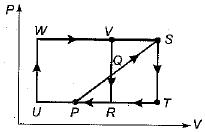Solution:
QUESTION: 15

A mixture of gases expands from 0.03 m3 to 0.06 m3 at a constant pressure of 1 MPa and absorbs 84 kJ of heat during the process. The change in internal energy of the mixture is

Solution: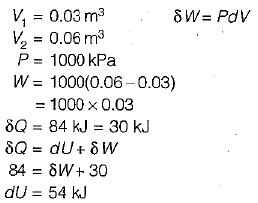Use Code STAYHOME200 and get INR 200 additional OFF Use Coupon Code

Track your progress, build streaks, highlight & save important lessons and more!

Similar Content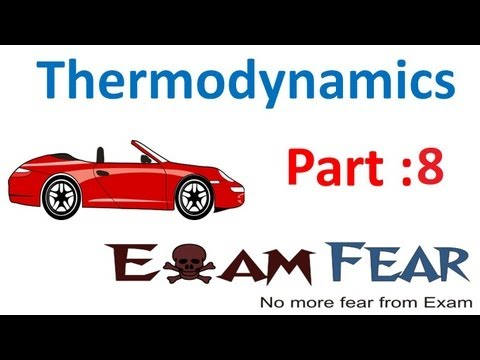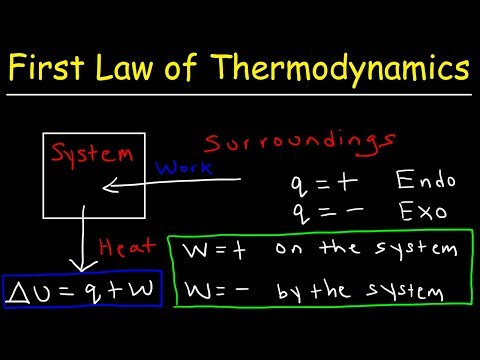Related tests